# Time Average Value of Pendulum String Tension

Mentor
Another member and I, in private conversations, have been discussing the time average tension in a pendulum string. He has done a numerical analysis of the problem, and his calculations indicate that the time average tension is less than mg. I have analyzed the problem analytically by expanding in the maximum angle ##\theta_0## for small values of this angle and found that the time average tension, at least for small maximum angles, is ##mg\left(1+\left(\frac{\theta_0}{2}\right)^2\right)##; so my analysis suggests that the time average tension is greater than mg. I'd like to give other members a chance to weigh in on this.

The parameters are: string length = L, mass = m, maximum angle with vertical = ##\theta_0##.

•dRic2 and wrobel

Staff Emeritus
maximum angle with vertical = θ

Surely that is the most critical parameter. At 90 degrees, the downward force is zero. With more than 90 degrees, it is negative. You analyzed it for small angles only. Might your friend have included larger angles?

Mentor
Surely that is the most critical parameter. At 90 degrees, the downward force is zero. With more than 90 degrees, it is negative. You analyzed it for small angles only. Might your friend have included larger angles?
My understanding is he solved it for a range of maximum angles.

Homework Helper
Gold Member
My understanding is he solved it for a range of maximum angles.
For the average to make sense, it must be over a full swing which then can be taken over half a swing by symmetry. Using a free body diagram and energy conservation you can show that the tension is given by $$T=3mg(\cos\theta -\cos\theta_0)$$ Then $$T_{\text{avg.}}=\frac{3mg\int_{-\theta_0}^{\theta_0}(\cos\theta -\cos\theta_0)d\theta}{\int_{-\theta_0}^{\theta_0} d\theta}.$$I don't have time to finish it now and plot it as a function of ##\theta_0##, but it should be easy to do.

•PhDeezNutz
Mentor
Are you averaging the magnitude of the tension or the vector?

Homework Helper
2022 Award
The time average of the vector tension over a cycle has got to be mg up, yes?

Mentor
For the average to make sense, it must be over a full swing which then can be taken over half a swing by symmetry. Using a free body diagram and energy conservation you can show that the tension is given by $$T=3mg(\cos\theta -\cos\theta_0)$$ Then $$T_{\text{avg.}}=\frac{3mg\int_{-\theta_0}^{\theta_0}(\cos\theta -\cos\theta_0)d\theta}{\int_{-\theta_0}^{\theta_0} d\theta}.$$I don't have time to finish it now and plot it as a function of ##\theta_0##, but it should be easy to do.
The other member and I both got $$T=mg(3\cos\theta -2\cos\theta_0)$$

Also, I said the time average not the average with respect to ##\theta##.

Also, we were referring the time average of the magnitude of the tension.

•hutchphd
Mentor
The time average of the vector tension over a cycle has got to be mg up, yes?
Yes. The vector average must be ##mg## up. I believe that in general the magnitude of the vector average will always be less than the average of the vector magnitude.

•sophiecentaur
Homework Helper
2022 Award
I think that true for the average of the square of the vector but the time average of the magnitude is not the sqrt of the time average of the magnitude squared. That was the wall I hit.

•Dale
Mentor
the time average of the magnitude is not the sqrt of the time average of the magnitude squared
Agreed, but I think that the triangle inequality still holds.

•jbriggs444
Mentor
At the bottom of the arc, the tension is greater than mg, right?

Homework Helper
2022 Award
Yes. But that's also where it is going fast. For larger theta as @anorlunda pointed out there will be a place where it can be less and it will spend more time there...so I'm not sure.
But clearly for small enough oscillations your result should hold for the cycle I think.

Mentor
The average with respect to theta is greater than mg, and increases with maximum angle; the approximation I derived for small angle agrees very closely with the exact average (wr theta) up to max angles of 45 degrees.

Homework Helper
Gold Member
The other member and I both got $$T=mg(3\cos\theta -2\cos\theta_0)$$

Also, I said the time average not the average with respect to ##\theta##.

Also, we were referring the time average of the magnitude of the tension.
I stand corrected. I impulsively thought that the pendulum can act as the time clock, but it can't.

Mentor
The average with respect to theta is greater than mg, and increases with maximum angle; the approximation I derived for small angle agrees very closely with the exact average (wr theta) up to max angles of 45 degrees.
The average with respect to theta is $$\frac{\bar{F}}{mg}=3\frac{\sin{\theta_0}}{\theta_0}-2\cos{\theta_0}$$which, at small values of ##\theta_0## becomes: $$\frac{\bar{F}}{mg}=1+\frac{\theta_0^2}{2}$$
At 30 degrees, the exact rhs is 1.13, compared to 1.14 from the approximation.

At 45 degrees, the exact rhs is 1.29, compared to 1.31 from the approximation.

The only question is whether the shorter residence times at smaller angles than at larger angles characteristic with time averaging is enough to reverse the effect.

Mentor
Based on the methodology in the following video,
I got the exact analytic solution for the time average tension in the string. This was based on the non-linear equation for the angular velocity:
$$\frac{d\theta}{dt}=\sqrt{\frac{2g}{L}}\sqrt{\cos{\theta}-\cos{\theta_0}}$$

My final result for the time average tension was $$\frac{\bar{F}}{mg}=3\left[2\frac{K(\sin{(\theta_0/2}))}{E(\sin{(\theta_0/2}))}-1\right]-2\cos{\theta_0}$$ where K and E are the first and second complete elliptic integral functions, respectively.

For a maximum angle of 30 degrees, I get 1.069 mg, compared to 1.069 mg from my approximate solution.

For a maximum angle of 60 degrees, I got 1.223 mg, compared to 1.274 mg from my approximate solution.

Last edited:
•alan123hk and hutchphd
Mentor
So I threw this into Mathematica to see what it says. I used the non-approximate differential equation:
$$\ddot \theta + \frac{g}{L} \sin(\theta) = 0$$ and the equation for the tension $$T=mg \cos(\theta)+ m (L \dot \theta)^2/L$$

I solved the differential equation with Mathematica and got $$\theta(t)=2 \text{am}\left(\frac{\dot \theta_0 t}{2},\frac{4g}{\dot \theta_0^2 L}\right)$$ where ##\theta (0)=0## and ##\dot \theta(0)=\dot \theta_0## and where ##\text{am}## is the Jacobi Amplitude function. I found it easier to parameterize by measuring ##t=0## when ##\theta=0## so that I could easily find the period using the "FindRoot" function. I used FindRoot to find ##t_f##, the time that the first period ends. Then I calculate the normalized average tension by $$\bar T = \frac{1}{mg t_f}\int_0^{t_f} |T| dt$$

In all of the below I used ##g=9.8## and ##L=m=1## and I varied ##\dot \theta_0##.

For ##\dot \theta_0=1## we see a nice approximately simple harmonic motion, with a period of ##t_f=2.02## and ##\bar T = 1.025##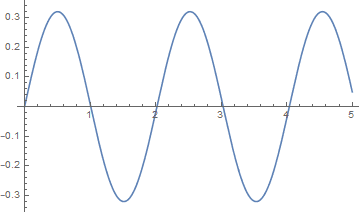For ##\dot \theta_0=6.1## we clearly see the expected noticeable deviation from simple harmonic motion, with a period of ##t_f=3.71## and ##\bar T = 1.606##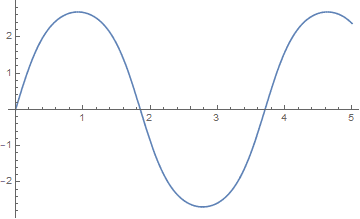For a maximum angle of 30 degrees, I get 1.069 mg, compared to 1.069 mg from my approximate solution.
I also got 1.07 for ##\dot \theta_0=1.620## which gets pretty close to 30 deg peak

For a maximum angle of 60 degrees, I got 1.223 mg, compared to 1.274 mg from my approximate solution.
I also got 1.223 for ##\dot \theta_0 = 3.13## which gets pretty close to 60 deg peak

Last edited:
•alan123hk, Ibix, Chestermiller and 1 other person
Mentor
So I threw this into Mathematica to see what it says. I used the non-approximate differential equation:
$$\ddot \theta + \frac{g}{L} \sin(\theta) = 0$$ and the equation for the tension $$T=mg \cos(\theta)+ m (L \dot \theta)^2/L$$

I solved the differential equation with Mathematica and got $$\theta(t)=2 \text{am}\left(\frac{\dot \theta_0 t}{2},\frac{4g}{\dot \theta_0^2 L}\right)$$ where ##\theta (0)=0## and ##\dot \theta(0)=\dot \theta_0## and where ##\text{am}## is the Jacobi Amplitude function. I found it easier to parameterize by measuring ##t=0## when ##\theta=0## so that I could easily find the period using the "FindRoot" function. I used FindRoot to find ##t_f##, the time that the first period ends. Then I calculate the normalized average tension by $$\bar T = \frac{1}{mg t_f}\int_0^{t_f} |T| dt$$

In all of the below I used ##g=9.8## and ##L=m=1## and I varied ##\dot \theta_0##.

For ##\dot \theta_0=1## we see a nice approximately simple harmonic motion, with a period of ##t_f=2.02## and ##\bar T = 1.025##

View attachment 282416
For ##\dot \theta_0=6.1## we clearly see the expected noticeable deviation from simple harmonic motion, with a period of ##t_f=3.71## and ##\bar T = 1.606##
View attachment 282417

I also got 1.07 for ##\dot \theta_0=1.620## which gets pretty close to 30 deg peak

I also got 1.223 for ##\dot \theta_0 = 3.13## which gets pretty close to 60 deg peak
Really nice work Dale! You're the man.

Thanks very much.

Chet

•Dale
Gold Member
The time average of the vector tension over a cycle has got to be mg up, yes?
If it were not mg'up' the mean position of the pendulum would have to be changing (up, down or sideways). That's Newton 1.
However, the long way round, due to @Dale and others is, of course, valid and it's a relief that both ways give the same answer.

•Dale and hutchphd
Mentor
Before concluding this thread, I thought it would be worthwhile showing the method I used to arrive at the analytic solution for the time averaged tension. The important feature is that the method did not actually require solving for the time variation of the pendulum angle.

The time average of the tension is given by: $$\bar{F}=\frac{\int_0^{T/4}{F(t)dt}}{(T/4)}$$where T is the period. In addition, we have that the angular velocity of the pendulum is expressed entirely as a function ##f(\theta)## of the pendulum angle ##\theta## as:$$\frac{d\theta}{dt}=\sqrt{\frac{2g}{L}}\sqrt{\cos{\theta}-\cos{\theta_0}}=f(\theta)$$Therefore, we can write $$dt=\frac{d\theta}{f(\theta)}$$where t runs from 0 to T/4 as ##\theta## runs from 0 to ##\theta_0##. In addition to this, we already know the tension as a function of ##\theta## as:$$F(\theta)=mg(3\cos\theta -2\cos\theta_0)$$Therefore, we can write: $$\bar{F}=\frac{\int_0^{\theta_0}{\frac{F(\theta)}{f(\theta)}d\theta}}{\int_0^{\theta_0}{\frac{d\theta}{f(\theta)}}}=mg\frac{\int_0^{\theta_0}{\frac{(3\cos\theta -2\cos\theta_0)}{\sqrt{\cos{\theta}-\cos{\theta_0}}}d\theta}}{\int_0^{\theta_0}{\frac{d\theta}{\sqrt{\cos{\theta}-\cos{\theta_0}}}}}$$
These integrals are evaluated analytically using the methods discussed in the video.

••alan123hk, hutchphd and Dale
Haborix
Based on the methodology in the following video,
I got the exact analytic solution for the time average tension in the string. This was based on the non-linear equation for the angular velocity:
$$\frac{d\theta}{dt}=\sqrt{\frac{2g}{L}}\sqrt{\cos{\theta}-\cos{\theta_0}}$$

My final result for the time average tension was $$\frac{\bar{F}}{mg}=3\left[2\frac{K(\sin{(\theta_0/2}))}{E(\sin{(\theta_0/2}))}-1\right]-2\cos{\theta_0}$$ where K and E are the first and second complete elliptic integral functions, respectively.

For a maximum angle of 30 degrees, I get 1.069 mg, compared to 1.069 mg from my approximate solution.

For a maximum angle of 60 degrees, I got 1.223 mg, compared to 1.274 mg from my approximate solution.

And if you substitute that exact result into WolframAlpha, it spits out a series expansion which matches your result in the first post.

Last edited by a moderator:
•Chestermiller
Based on the methodology in the following video
Nice talk. He told everything except for the sole that should have been told: the phase portrait.

Sorry for offtop

•etotheipi
alan123hk
I tried to use a spreadsheet for numerical analysis. A total of 20,000 calculation steps were used, but my computer only took less than a second to complete the calculation.
When the maximum angle is 60 degrees, I found that the average tension is 1.223 mg, which is greater than 1.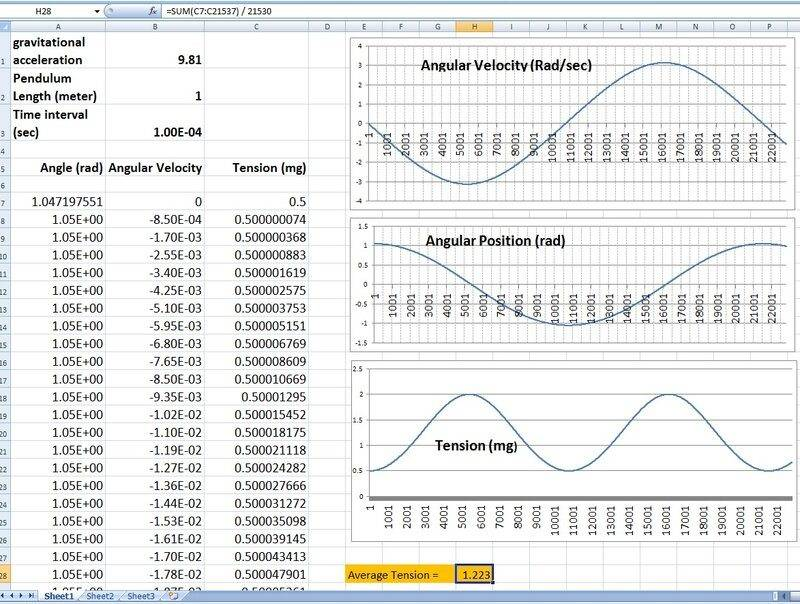Last edited:
•Dale and sophiecentaur
Gold Member
but my computer only took less than a second to complete the calculation.
It just goes to show how processor-intensive modern video games are. That is probably the highest demand from most domestic users. A few simple 'sums' are no trouble for your PC, even though a spreadsheet plods through those things using interpretive routines without the help of a compiled language.

•alan123hk
alan123hk
Because I haven't used this kind of numerical analysis for a long time, there are many imperfections in the calculations I uploaded before.

By averaging only a quarter period and other optimization measures, I now improve the efficiency of the calculation method, as shown below

1. The time interval can be increased from 0.1 ms to 1.0 ms
2. The number of iterations dropped sharply from 20000 to only about 1000

Please note that the calculation result only increased from 1.223 to 1.224, with an error of less than 0.1%, which really surprised me.

I used the second-order Runge–Kutta method, if anyone is interested, you can refer to this link
https://en.wikipedia.org/wiki/Runge–Kutta_methods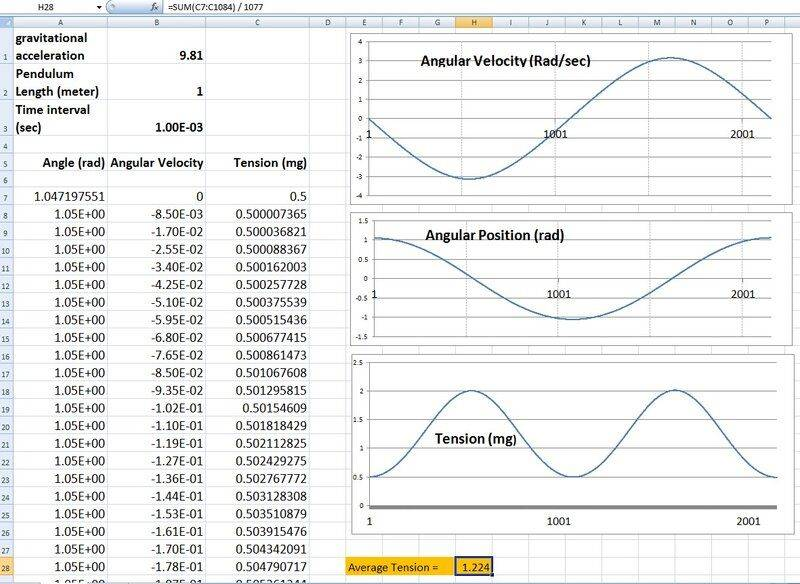•hutchphd and Dale
cmb
I think you can discretise the SHM into horizontal and vertical components.

I struggle to imagine the average of the vertical is not mg? There is no net work being done. Just a consideration of 'energy' puts that one to bed, doesn't it? Am I under-thinking that one?

The horizontal should be quite easy to work out, equation of SHM within a given displacement and a given period.

So total tension will always be the quadrature sum of the vertical (average mg) plus the horizontal restoring SHM force.

I think? No more complicated than that?

Gold Member
I think you can discretise the SHM into horizontal and vertical components.

I struggle to imagine the average of the vertical is not mg? There is no net work being done. Just a consideration of 'energy' puts that one to bed, doesn't it? Am I under-thinking that one?

The horizontal should be quite easy to work out, equation of SHM within a given displacement and a given period.

So total tension will always be the quadrature sum of the vertical (average mg) plus the horizontal restoring SHM force.

I think? No more complicated than that?
Isn’t it obvious that the mean force has to be mg or 1. The string would have to be changing length or 2. The suspension point would have to be moving.
If not, I think we could be into a reactionless drive system.

Mentor
Isn’t it obvious that the mean force has to be mg or 1. The string would have to be changing length or 2. The suspension point would have to be moving.
If not, I think we could be into a reactionless drive system.
It is obvious. But they are calculating the mean of the magnitude of the force which is larger.

•sophiecentaur and hutchphd
Homework Helper
2022 Award
Yes as mentioned in #6 to be sure we were on the same page:

The time average of the vector tension over a cycle has got to be mg

Is there an easy (not involving looking up elliptic functions although that was well done) to answer the original question About time average of T definitively (is it greater than mg) ? Something involving the triangle inequality and positive definiteness? I can't seem to pin it down, and it is niggling at me.

•sophiecentaur
Gold Member
It is obvious. But they are calculating the mean of the magnitude of the force which is larger.
Perhaps the mean is not the right sort of 'average' to be using. The appropriate 'rules' for vectors need to be followed if the result of a calculation is to be meaningful. N1 has to be followed.
That triangle inequality must be the relevant factor, I think.
(A1 + A2)2 + (B1 + B2)2 is not equal to
A12+A22+B12+B22
Bad notation but it shows what I mean. It agrees with @Dale way back in the thread.

•Dale
alan123hk
I have a thought in my mind, that is, when the initial angle is greater than 90 degrees, whether the average tension will always be less than 1mg. After some effort, if there is no error in my calculation, I finally found that for example, the initial angle is 175 degrees, the average tension will be much less than 1mg. I believe that when the angle is closer to 180 degrees, the average tension may even gradually approach zero.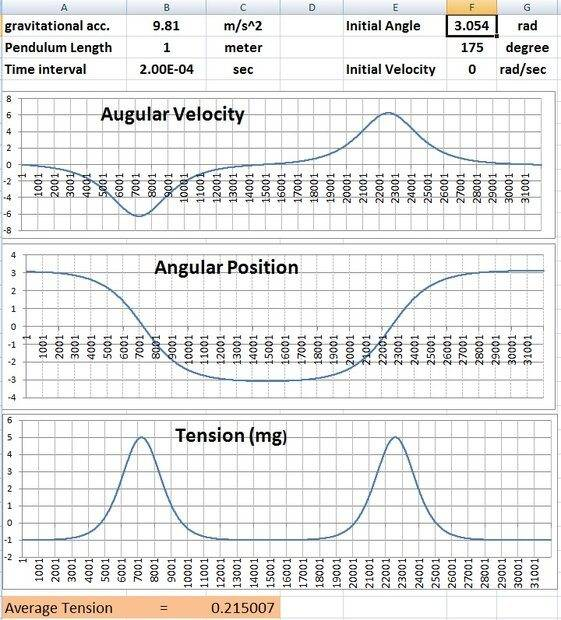By the way, I am considering the practical application of the calculation of this tension time average. One of the possible applications is that the probability of deformation and breaking or the service life of the rigid inflexible rod of the pendulum is proportional to the average tension. But I don't know if I should use the time average of absolute values in this case.

Last edited:
cmb
Isn’t it obvious that the mean force has to be mg or 1. The string would have to be changing length or 2. The suspension point would have to be moving.
If not, I think we could be into a reactionless drive system.
Vertically yes, that was my point.

But in the horizontal there has to be a

cmb
Let me please ask a different question that might hold the same answer: Let's consider increasing the angular displacement of the pendulum in our calculation until it approaches pi (we can also consider exceeding pi?);-

Let's assume the pendulum is a stiff inflexible rod of negligible mass.

At some operational point as the pendulum motion approaches pi, the tension in the rod will go to zero at some point on the upward swing and reverse to compression, according to the mass of the pendulum bob, m.

As it just reaches the top of the swing and increasingly balances before going 'over the top', the 'time average' tension will clearly approach negative mg, -mg, because it may balance for a long time at the top of the swing. It spends longer at high angular displacements than at small ones.

Ergo, if the force is time averaged (by dt), it is probably a lower average tension generally, and will tend to zero and even negative (compression) in some conditions (imagine it balances exceeedingly close to pi for a long time before swinging back). If it is averaged according to angle, a (i.e. averaged by da), it will clearly be a completely different answer.

I would argue, independent of maths, the thought experiment above suggests the angle of excursion of the pendulum clearly makes a difference to the answer, and deciding what you are integrating the tension against (angle or time) likewise requires resolution before there is any satisfactory answer.

Staff Emeritus
Gold Member
Yes as mentioned in #6 to be sure we were on the same page:

Is there an easy (not involving looking up elliptic functions although that was well done) to answer the original question About time average of T definitively (is it greater than mg) ? Something involving the triangle inequality and positive definiteness? I can't seem to pin it down, and it is niggling at me.

If ##T_x(t)## is the tension in the x direction at time t, and similar for ##T_y(t)##, and one full period has time ##p##, then the average tension is

$$\frac{1}{p} \int_0^p \sqrt{T_x^2+T_y^2} dt > \frac{1}{p} \int_0^p \sqrt{T_y^2} dt = \frac{1}{p} \int_0^p |T_y| dt \geq mg$$

The first strict inequality comes from the fact that ##T_x## is almost everywhere non-zero, and the second inequality comes from the fact that the signed average y tension is mg, so if e.g. the pendulum does full rotations you might get a strict inequality.

Last edited: